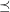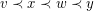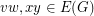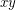# Blankenship, Robin

## Book Thickness of Subdivisions ★★

Author(s): Blankenship; Oporowski

Letbe a finite undirected simple graph.

A-page book embedding ofconsists of a linear orderofand a (non-proper)-colouring ofsuch that edges with the same colour do not cross with respect to. That is, iffor some edges, thenandreceive distinct colours.

One can think that the vertices are placed along the spine of a book, and the edges are drawn without crossings on the pages of the book.

The book thickness of, denoted by btis the minimum integerfor which there is a-page book embedding of.

Letbe the graph obtained by subdividing each edge ofexactly once.

Conjecture   There is a functionsuch that for every graph,Keywords: book embedding; book thickness